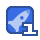# 第十一节 图像处理之霍夫检测直线229收藏

import cv2 as cv import numpy as np #直线检测 #使用霍夫直线变换做直线检测，前提条件：边缘检测已经完成 #标准霍夫线变换 def line_detection(image): gray = cv.cvtColor(image, cv.COLOR_RGB2GRAY) edges = cv.Canny(gray, 50, 150) #apertureSize参数默认其实就是3 cv.imshow("edges", edges) #cv.HoughLines参数设置：参数1，灰度图像；参数二，以像素为单位的距离精度（一般都是1，进度高，但是速度会慢一点） #参数三，以弧度为单位的角度精度（一般是1rad）；参数四，阈值，大于阈值threshold的线段才可以被检测通过并返回到结果中 #该函数返回值为rho与theta lines = cv.HoughLines(edges, 1, np.pi/180, 200) for line in lines: rho, theta = line #line存储的是点到直线的极径和极角，其中极角是弧度表示的。 a = np.cos(theta) #theta是弧度 b = np.sin(theta) x0 = a * rho #代表x = r * cos（theta） y0 = b * rho #代表y = r * sin（theta） x1 = int(x0 + 1000 * (-b)) #计算直线起点横坐标 y1 = int(y0 + 1000 * a) #计算起始起点纵坐标 x2 = int(x0 - 1000 * (-b)) #计算直线终点横坐标 y2 = int(y0 - 1000 * a) #计算直线终点纵坐标 注：这里的数值1000给出了画出的线段长度范围大小，数值越小，画出的线段越短，数值越大，画出的线段越长 cv.line(image, (x1, y1), (x2, y2), (0, 0, 255), 2) #点的坐标必须是元组，不能是列表。 cv.imshow("image-lines", image) #统计概率霍夫线变换 def line_detect_possible_demo(image): gray = cv.cvtColor(image, cv.COLOR_RGB2GRAY) edges = cv.Canny(gray, 50, 150, apertureSize=3) # apertureSize参数默认其实就是3 lines = cv.HoughLinesP(edges, 1, np.pi / 180, 60, minLineLength=60, maxLineGap=5) for line in lines: x1, y1, x2, y2 = line cv.line(image, (x1, y1), (x2, y2), (0, 0, 255), 2) cv.imshow("line_detect_possible_demo",image) src = cv.imread("E:/opencv/picture/track.jpg") print(src.shape) cv.namedWindow('input_image', cv.WINDOW_AUTOSIZE) cv.imshow('input_image', src) line_detection(src) src = cv.imread("E:/opencv/picture/track.jpg") #调用上一个函数后，会把传入的src数组改变，所以调用下一个函数时，要重新读取图片 line_detect_possible_demo(src) cv.waitKey(0) cv.destroyAllWindows() 霍夫检测直线原理： 关于hough变换，核心以及难点就是关于就是有原始空间到参数空间的变换上。以直线检测为例，假设有一条直线L，原点到该直线的垂直距离为p，垂线与x轴夹角为θθ，那么这条直线是唯一的，且直线的方程为 ρ=xcosθ+ysinθρ=xcosθ+ysinθ, 如下图所

...展开详情

• 23
资源
• 2
粉丝
•等级
•分享宗师第十一节 图像处理之霍夫检测直线 26积分/C币 立即下载
1/0